Next: Ring Current Up: Charged Particle Motion Previous: Magnetic Mirrors

Plasma confinement via magnetic mirroring occurs in nature as well as in unsuccessful fusion devices. For instance, the Van Allen radiation belts, which surround the Earth, consist of energetic particles trapped in the Earth's dipole-like magnetic field. These belts were discovered by James A. Van Allen and co-workers using data taken from Geiger counters which flew on the early U.S. satellites, Explorer 1 (which was, in fact, the first U.S. satellite), Explorer 4, and Pioneer 3. Van Allen was actually trying to measure the flux of cosmic rays (high energy particles whose origin is outside the Solar System) in outer space, to see if it was similar to that measured on Earth. However, the flux of energetic particles detected by his instruments so greatly exceeded the expected value that it prompted one of his co-workers to exclaim, My God, space is radioactive!'' It was quickly realized that this flux was due to energetic particles trapped in the Earth's magnetic field, rather than to cosmic rays.

There are, in fact, two radiation belts surrounding the Earth. The inner belt, which extends from about 1-3 Earth radii in the equatorial plane is mostly populated by protons with energies exceeding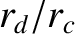MeV. The origin of these protons is thought to be the decay of neutrons which are emitted from the Earth's atmosphere as it is bombarded by cosmic rays. The inner belt is fairly quiescent. Particles eventually escape due to collisions with neutral atoms in the upper atmosphere above the Earth's poles. However, such collisions are sufficiently uncommon that the lifetime of particles in the belt range from a few hours to 10 years. Clearly, with such long trapping times only a small input rate of energetic particles is required to produce a region of intense radiation.

The outer belt, which extends from about 3-9 Earth radii in the equatorial plane, consists mostly of electrons with energies belowMeV. The origin of these electrons is via injection from the outer magnetosphere. Unlike the inner belt, the outer belt is very dynamic, changing on time-scales of a few hours in response to perturbations emanating from the outer magnetosphere.

In regions not too far distant (i.e., less than 10 Earth radii) from the Earth, the geomagnetic field can be approximated as a dipole field,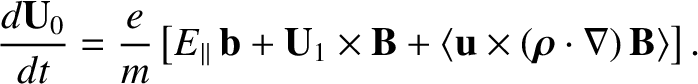(121)

where we have adopted conventional spherical polar coordinates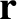aligned with the Earth's dipole moment, whose magnitude is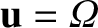. It is usually convenient to work in terms of the latitude,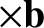, rather than the polar angle,. An individual magnetic field-line satisfies the equation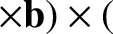(122)

where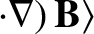is the radial distance to the field-line in the equatorial plane (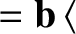). It is conventional to label field-lines using the L-shell parameter,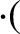. Here,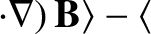is the Earth's radius. Thus, the variation of the magnetic field-strength along a field-line characterized by a given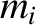-value is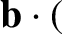(123)

where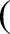is the equatorial magnetic field-strength on the Earth's surface.

Consider, for the sake of simplicity, charged particles located on the equatorial plane () whose velocities are predominately directed perpendicular to the magnetic field. The proton and electron gyrofrequencies are written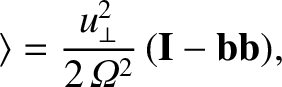(124)

and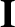(125)

respectively. The proton and electron gyroradii, expressed as fractions of the Earth's radius, take the form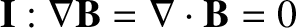(126)

and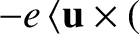(127)

respectively. It is clear that MeV energy charged particles in the inner magnetosphere (i.e,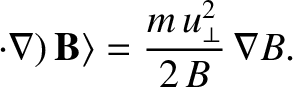) gyrate at frequencies which are much greater than the typical rate of change of the magnetic field (which changes on time-scales which are, at most, a few minutes). Likewise, the gyroradii of such particles are much smaller than the typical variation length-scale of the magnetospheric magnetic field. Under these circumstances, we expect the magnetic moment to be a conserved quantity: i.e., we expect the magnetic moment to be a good adiabatic invariant. It immediately follows that any MeV energy protons and electrons in the inner magnetosphere which have a sufficiently large magnetic moment are trapped on the dipolar field-lines of the Earth's magnetic field, bouncing back and forth between mirror points located just above the Earth's poles.

It is helpful to define the pitch-angle,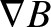(128)

of a charged particle in the magnetosphere. If the magnetic moment is a conserved quantity then a particle of fixed energy drifting along a field-line satisfies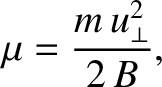(129)

where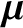is the equatorial pitch-angle (i.e., the pitch-angle on the equatorial plane) and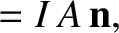is the magnetic field-strength on the equatorial plane. It is clear from Eq. (123) that the pitch-angle increases (i.e., the parallel component of the particle velocity decreases) as the particle drifts off the equatorial plane towards the Earth's poles.

The mirror points correspond to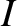(i.e.,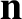). It follows from Eqs. (123) and (129) that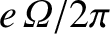(130)

where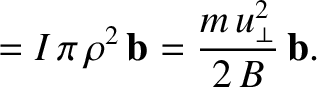is the magnetic field-strength at the mirror points, and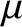is the latitude of the mirror points. Clearly, the latitude of a particle's mirror point depends only on its equatorial pitch-angle, and is independent of the-value of the field-line on which it is trapped.

Charged particles with large equatorial pitch-angles have small parallel velocities, and mirror points located at relatively low latitudes. Conversely, charged particles with small equatorial pitch-angles have large parallel velocities, and mirror points located at high latitudes. Of course, if the pitch-angle becomes too small then the mirror points enter the Earth's atmosphere, and the particles are lost via collisions with neutral particles. Neglecting the thickness of the atmosphere with respect to the radius of the Earth, we can say that all particles whose mirror points lie inside the Earth are lost via collisions. It follows from Eq. (130) that the equatorial loss cone is of approximate width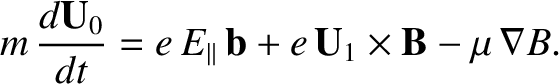(131)

where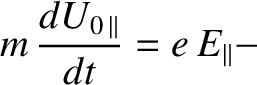is the latitude of the point where the magnetic field-line under investigation intersects the Earth. Note that all particles with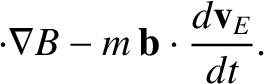and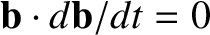lie in the loss cone. It is easily demonstrated from Eq. (122) that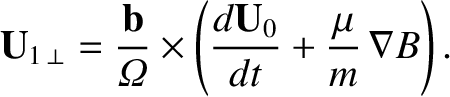(132)

It follows that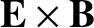(133)

Thus, the width of the loss cone is independent of the charge, the mass, or the energy of the particles drifting along a given field-line, and is a function only of the field-line radius on the equatorial plane. The loss cone is surprisingly small. For instance, at the radius of a geostationary orbit (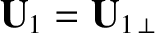), the loss cone is less than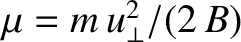degrees wide. The smallness of the loss cone is a consequence of the very strong variation of the magnetic field-strength along field-lines in a dipole field--see Eqs. (120) and (123).

A dipole field is clearly a far more effective configuration for confining a collisionless plasma via magnetic mirroring than the more traditional linear configuration shown in Fig. 1. In fact, M.I.T. has recently constructed a dipole mirror machine. The dipole field is generated by a superconducting current loop levitating in a vacuum chamber.

The bounce period,, is the time it takes a particle to move from the equatorial plane to one mirror point, then to the other, and then return to the equatorial plane. It follows that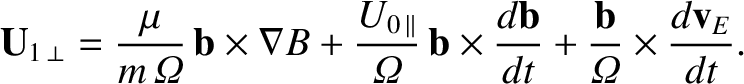(134)

where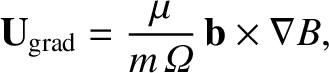is an element of arc length along the field-line under investigation, and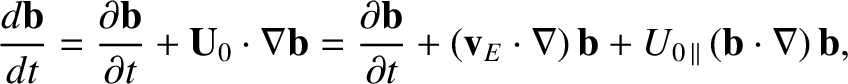. The above integral cannot be performed analytically. However, it can be solved numerically, and is conveniently approximated as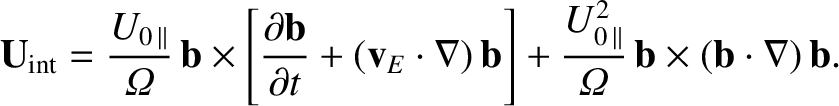(135)

Thus, for protons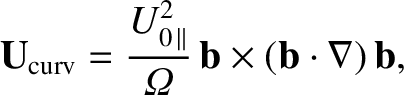(136)

whilst for electrons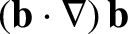(137)

It follows that MeV electrons typically have bounce periods which are less than a second, whereas the bounce periods for MeV protons usually lie in the range 1 to 10 seconds. The bounce period only depends weakly on equatorial pitch-angle, since particles with small pitch angles have relatively large parallel velocities but a comparatively long way to travel to their mirror points, and vice versa. Naturally, the bounce period is longer for longer field-lines (i.e., for larger).Next: Ring Current Up: Charged Particle Motion Previous: Magnetic Mirrors
Richard Fitzpatrick 2011-03-31